Addition and Subtraction Facts Drill Within 10

Times up!
Times up! You may finish answering the current question and then proceed to your score!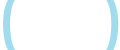Sorry, you have used all of your available hints for this lesson.
Practice Limit Reached

You've reached your daily practice limit of 25 questions.

(Must be 18 years old to sign up.)

Are you over 18 years old?
Award InformationAwarded toQuestion HintChoose Family Member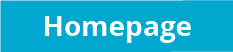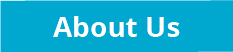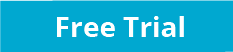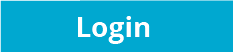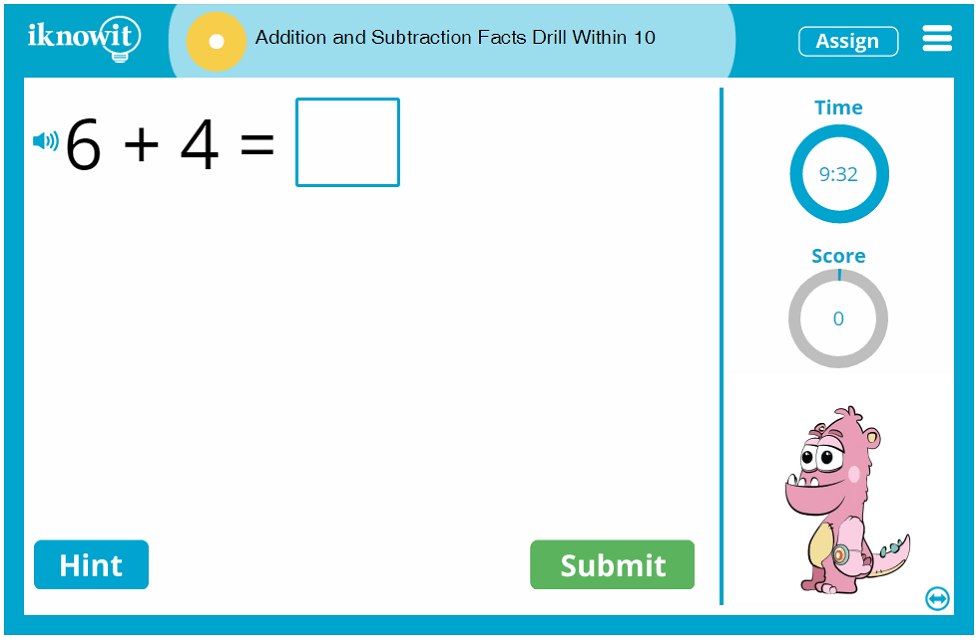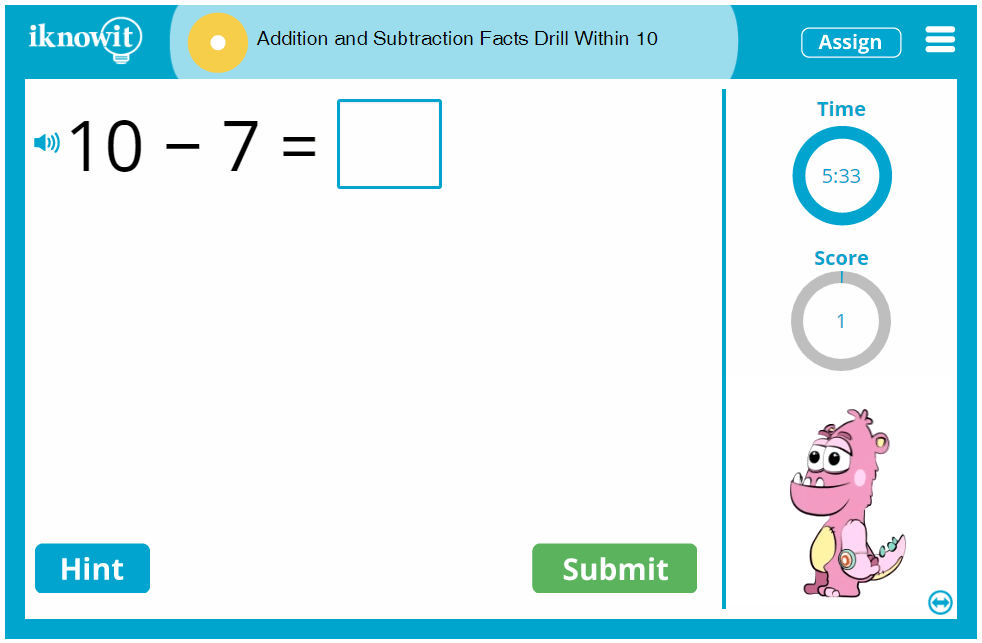Interactive Math Practice - Addition and Subtraction Facts Drill within Ten

It's time to practice basic addition and subtraction facts within ten! This first grade-level lesson will help your students become more confident and accurate in their basic addition and subtraction skills. All addition and subtraction facts in this online math lesson feature addends, minuends, and subtrahends within ten.

In this drill-style lesson, math problems are presented in a horizontal format. Students will fill in the blank with the correct answer. If students need a little extra help, they can click on the hint button, which will show them a picture of dice to help them visualize the math problem. They can also click the speaker icon in the upper-left corner of their practice screen to have the question read aloud to them in a clear voice. (This is a great option for ESL/ELL students, or students who prefer auditory learning.) If students answer a question incorrectly, a detailed explanation page will appear with a similar visual representation as the hint, showing them how the addition or subtraction problem should be solved. This way, students can learn from their mistakes and improve their skills throughout their practice session.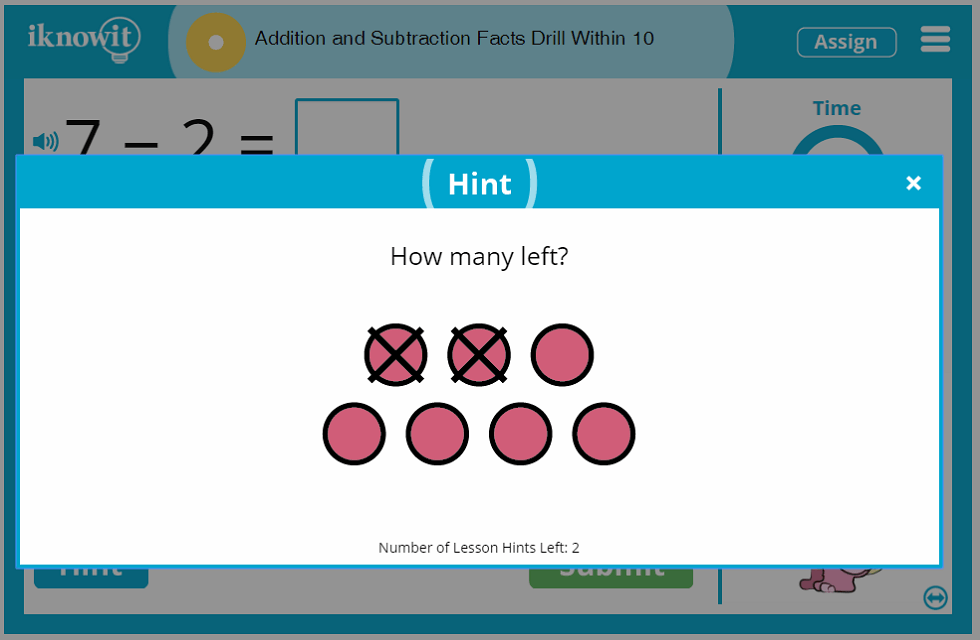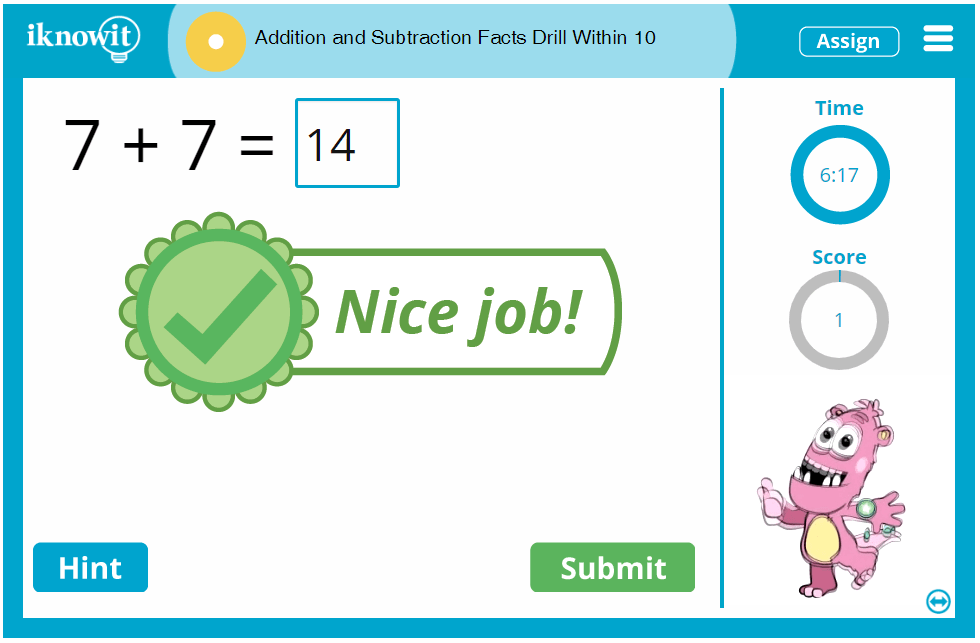Dive Deeper into Math Practice with I Know It

Students really enjoy the time they spend practicing math skills with the 'I Know It' math program. After all, our goal is to provide interactive math practice that's both educational and fun! While students find their math practice sessions fun, yet challenging, teachers appreciate the wide range of math topics we cover in each grade level, as well as the quality of our math lessons. Each lesson has a progress tracker to show children how many questions they've answered so far in the lesson, as well as a score tracker to show students how many problems they've solved correctly so far. We encourage you to try out our kid-friendly, easy-to-navigate website with your class today! Try out this addition and subtraction facts drill within ten with your class! Be sure to browse our complete collection of other math topics too!

Sign up for a free sixty-day trial of 'I Know It' so you can try out this basic addition and subtraction math practice session with your students today! Your class may practice for free, but they will be capped at a total of twenty-five math problems per day across all 'I Know It' math lessons. For unlimited access to 'I Know It', its content, and administrative features, you will need to become a member of the website.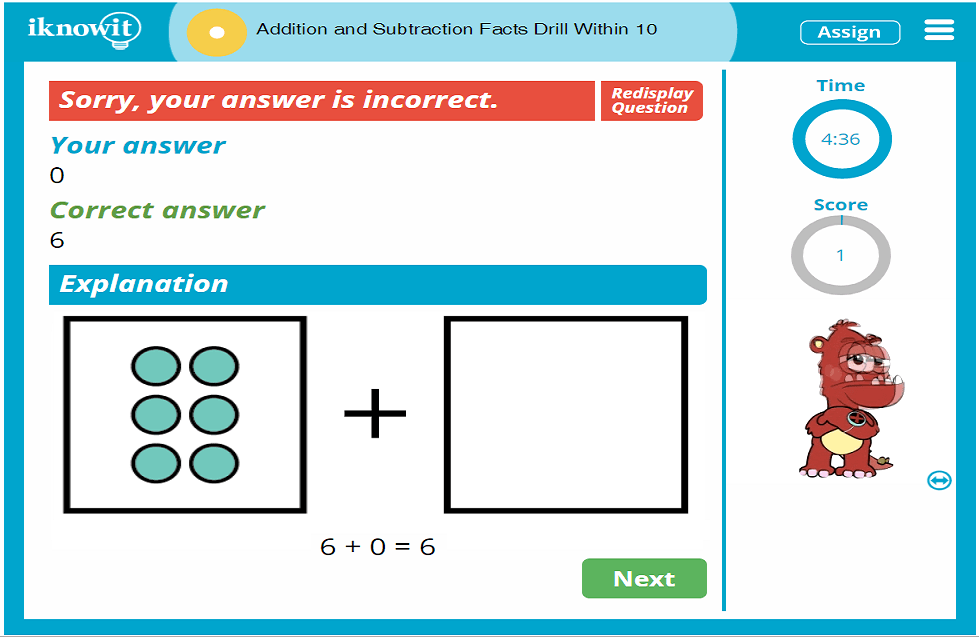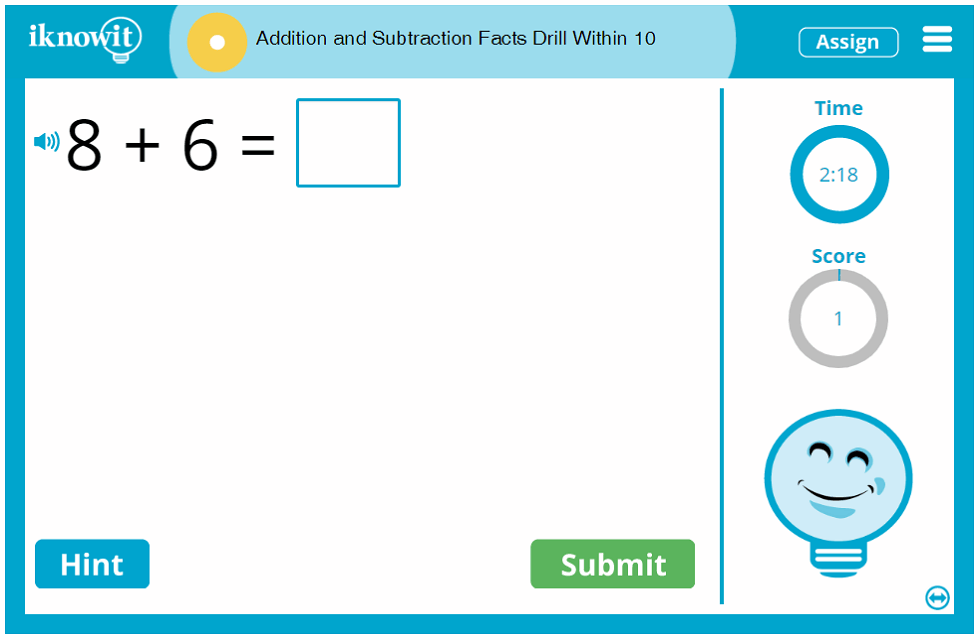Level

This online math lesson is categorized at a 'Level A.' It may be ideal for first grade students.

Common Core Standard

1.OA.6
Operations and Algebraic Thinking

Students will be able to add and subtract within twenty, demonstrating fluency for addition and subtraction within ten. Students should be able to use strategies including: counting on; making ten; decomposing a number leading to a ten; using the relationship between addition and subtraction; and creating equivalent but easier or known sums.

You might also be interested in...

Add and Subtract Within 20 (Level A)
In this interactive math lesson for first grade, children will practice adding and subtracting within twenty. Math problems are presented in a variety of formats.

Bar Models (Addition and Subtraction within 20) (Level A)
Students will use bar models to practice addition and subtraction within twenty in this online math lesson for first grade. Math problems are presented in a variety of formats.